### Percentage 'of'

Frequently, we need to express one quantity as a percentage of another.

Here is an example where we colour different percentages of a set of 20 stars.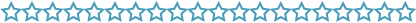1. If 100% of the stars are to be orange,
\begin{align}100\%\ \text{of}\ 20&=\dfrac{100}{100}×20\\\\ &=1×20\\\\ &=20\end{align} We colour 20 out of the 20 stars.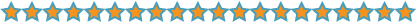100% of 20 is 20.
2. If 50% of the stars are to be orange,
\begin{align}50\%\ \text{of}\ 20&=\dfrac{50}{100}×20\\\\ &=\dfrac{1}{2}×\dfrac{20}{1}\\\\ &=\dfrac{20}{2}\\\\ &=10\end{align} We colour ten out of the 20 stars.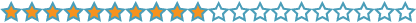50% of 20 is ten.

3. If 10% of the stars are to be orange,
\begin{align}10\%\ \text{of}\ 20 &=\dfrac{10}{100}×20\\\\      &=\dfrac{1}{10}×\dfrac{20}{1}\\\\      &=\dfrac{20}{10}\\\\       &=2\end{align}We colour two out of the 20 stars.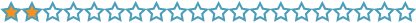10% of 20 is two.
4. If 5% of the stars are to be orange,
\begin{align}5\%\ \text{of}\ 20&=\dfrac{5}{100}×20\\\\   &=\dfrac{1}{20}×\dfrac{20}{1}\\\\   &=\dfrac{20}{20}\\\\   &=1\end{align} We colour one out of the 20 stars.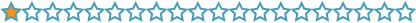5% of 20 is one.
5. If 35% of the stars are to be orange,
\begin{align}35\%\ \text{of}\ 20&=\dfrac{35}{100}×20\\\\      &=\dfrac{35}{100}×\dfrac{20}{1}\\\\       &=\dfrac{35}{5}×\dfrac{1}{1}\\\\         &=7\end{align} We colour seven out of 20 stars.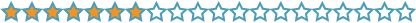35% of 20 is seven.

### Summary

To calculate the percentage of an amount, we convert the percentage to a fraction and multiply. The word 'of' tells us to multiply fractions.